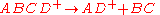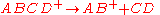xUnimolecular ion decompositionEncyclopedia
Unimolecular ion decomposition is the fragmentation of a gas phase ion in a reaction with a molecularity
Molecularity
Molecularity in chemistry is the number of colliding molecular entities that are involved in a single reaction step. While the order of a reaction is derived experimentally, the molecularity is a theoretical concept and can only be applied to elementary reactions...

of one. Ions with sufficient internal energy
Internal energy
In thermodynamics, the internal energy is the total energy contained by a thermodynamic system. It is the energy needed to create the system, but excludes the energy to displace the system's surroundings, any energy associated with a move as a whole, or due to external force fields. Internal...

may fragment in a mass spectrometer, which in some cases may degrade the mass spectrometer performance, but in other cases, such as tandem mass spectrometry
Tandem mass spectrometry
Tandem mass spectrometry, also known as MS/MS or MS2, involves multiple steps of mass spectrometry selection, with some form of fragmentation occurring in between the stages.-Tandem MS instruments:...

, the fragmentation can reveal information about the structure of the ion.

## Wahrhaftig diagram

A Wahrhaftig diagram illustrates the relative contributions in unimolecular ion decomposition of direct fragmentation and fragmentation following rearrangement. The x-axis of the digram represents the internal energy of the ion. The lower part of the diagram shows the logarithm
Logarithm
The logarithm of a number is the exponent by which another fixed value, the base, has to be raised to produce that number. For example, the logarithm of 1000 to base 10 is 3, because 1000 is 10 to the power 3: More generally, if x = by, then y is the logarithm of x to base b, and is written...

of the rate constant k for unimolecular dissociation] whereas the upper portion of the diagram indicates the probability of forming a particular product ion. The green trace in the lower part of the diagram indicates the rate of the rearrangement reacton given byand the blue trace indicates the direct cleavage reactionA rate constant of 106 s-1 is sufficiently fast for ion decomposition within the ion source
Ion source
An ion source is an electro-magnetic device that is used to create charged particles. These are used primarily to form ions for mass spectrometers, optical emission spectrometers, particle accelerators, ion implanters and ion engines.- Electron ionization :...

of a typical mass spectrometer. Ions with rate constants less than 106 s-1 and greater than approximately 105 s-1 (lifetimes between 105 and 106 s) have a high probability of decomposing in the mass spectrometer between the ion source and the detector. These rate constants are indicated in the Wahrhaftig diagram by the log k = 5 and log k = 6 dashed lines.

Indicated on the rate constant plot are the reaction critical energy (also called the activation energy
Activation energy
In chemistry, activation energy is a term introduced in 1889 by the Swedish scientist Svante Arrhenius that is defined as the energy that must be overcome in order for a chemical reaction to occur. Activation energy may also be defined as the minimum energy required to start a chemical reaction...

) for the formation of AD+, E0(AD+) and AB+, E0(AB+). These represent the minimum internal energy of ABCD+ required to form the respective product ions: the difference in the zero point energy of ABCD+ and that of the activated complex
Activated complex
In chemistry an activated complex is defined by the International Union of Pure and Applied Chemistry as "that assembly of atoms which corresponds to an arbitrary infinitesimally small region at or near the col of a potential energy surface"...

.

When the internal energy of ABCD+ is greater than Em(AD+), the ions are metastable (indicated by m*); this occurs near log k > 5. A metastable ion has sufficient internal energy
Internal energy
In thermodynamics, the internal energy is the total energy contained by a thermodynamic system. It is the energy needed to create the system, but excludes the energy to displace the system's surroundings, any energy associated with a move as a whole, or due to external force fields. Internal...

to dissociate prior to detection. The energy Es(AD+) is defined as the internal energy of ABCD+ that results in an equal probability that ABCD+and AD+ leave the ion source, which occurs at near log k = 6. When the precursor ion has an internal energy equal to Es(AB+), the rates of formation of AD+ and AB+ are equal.

## Thermodynamic and kinetic effects

Like all chemical reactions, the unimolecular decomposition of ions is subject to thermodynamic versus kinetic reaction control: the kinetic product forms faster, whereas the thermodynamic product is more stable. In the decomposition of ABCD+, the reaction to form AD+ is thermodynamically favored and the reaction to form AB+is kinetically favored. This is because the AD+ reaction has favorable enthalpy
Enthalpy
Enthalpy is a measure of the total energy of a thermodynamic system. It includes the internal energy, which is the energy required to create a system, and the amount of energy required to make room for it by displacing its environment and establishing its volume and pressure.Enthalpy is a...

and the AB+ has favorable entropy
Entropy
Entropy is a thermodynamic property that can be used to determine the energy available for useful work in a thermodynamic process, such as in energy conversion devices, engines, or machines. Such devices can only be driven by convertible energy, and have a theoretical maximum efficiency when...

.

In the reaction depicted schematically in the figure, the rearrangement reaction forms a double bond B=C and a new single bond A-D, which offsets the cleavage of the A-B and C-D bonds. The formation of AB+ requires bond cleavage without the offsetting bond formation. However, the steric effect makes it more difficult for the molecule to achieve the rearrangement transition state and form AD+. The activated complex with strict steric requirements is referred to as a "tight complex" whereas the transition state without such requirements is called a "loose complex."

• Metastability
Metastability
Metastability describes the extended duration of certain equilibria acquired by complex systems when leaving their most stable state after an external action....

• Transition state theory
Transition state theory
Transition state theory explains the reaction rates of elementary chemical reactions. The theory assumes a special type of chemical equilibrium between reactants and activated transition state complexes....

• RRKM theory
RRKM theory
The Rice–Ramsperger–Kassel–Marcus theory of chemical reactivity was developed by Rice and Ramsperger in 1927 and Kassel in 1928 . The RRK theory was generalized into the RRKM theory in 1952 by Marcus who took the transition state theory developed by Eyring in 1935 into account...

• Tandem mass spectrometry
Tandem mass spectrometry
Tandem mass spectrometry, also known as MS/MS or MS2, involves multiple steps of mass spectrometry selection, with some form of fragmentation occurring in between the stages.-Tandem MS instruments:...